# Unlock the Secrets of Upper Level Math with Expert Guidance and Real-World Applications

Recent questions in Upper Level Mathkirstenf9335jv 2023-02-24

## If two angles of two triangles are congruent to each other, then the third angles will be congruent to each other. Using the above statement, choose which two congruency criteria eventually becomes same. A)SAS and ASA; B)AAS and SAS; C)AAS and ASA; D) No two congruency criteria are sameVarceprewN3M 2022-11-25

## Evaluate $6{C}_{3}$pancho ono2022-11-16

##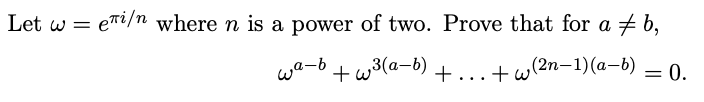Upper Level MathOpen questionCarlos Manuel Ordoñez2022-11-14

## Solve the following differential equation dx/dy=-[(4y2+6xy)/(3y2+2x)]Messiah Sutton 2022-11-09

## The velocity distribution for laminar flow between parallel plates is given by:$\frac{u}{{u}_{max}}=1-{\left(\frac{2y}{h}\right)}^{2}$where h is the distance separating the plates and the origin is placed midway between the plates. Consider a flow of water at ${15}^{\circ }C$ , with ${u}_{max}=0.30\frac{m}{sec}$ and . Calculate the shear stress on the upper plate and give its direction.

Upper Level MathOpen questionpancho ono2022-10-29

##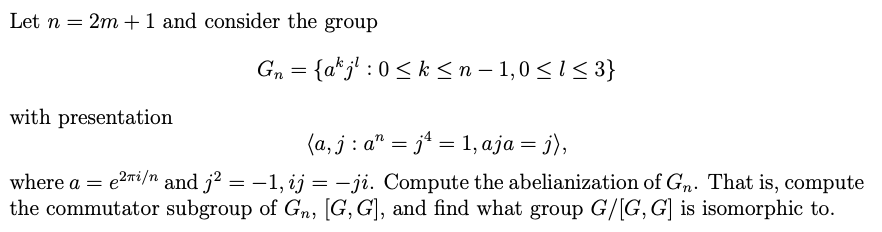Upper Level MathOpen questionBenu Sharma2022-10-28

## In August 2013, E*TRADE Financial was offering only 0.05% interest on its online checking accounts, with interest reinvested monthly. Find the associated exponential model for the value of a \$5000 deposit after "t" years. Assuming that this rate of return continued for 7 years, how much would a deposit of \$5000 in August 2013 be worth in August 2020? (Answer to the nearest \$1. )

Upper Level MathOpen questionpancho ono2022-10-26

##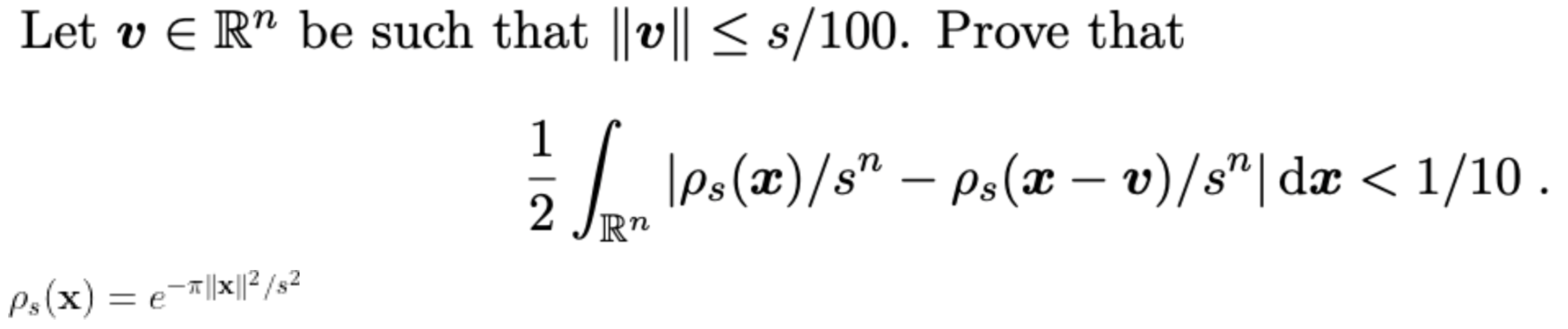Upper Level MathOpen questionpancho ono2022-10-26

##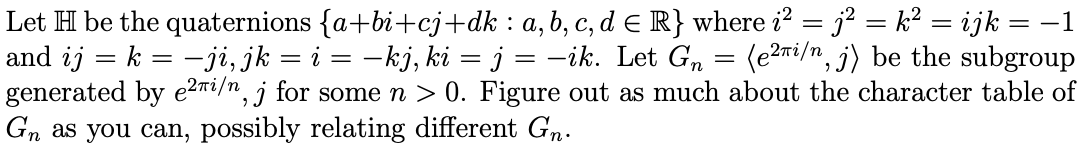Upper Level MathOpen questionpancho ono2022-10-26

##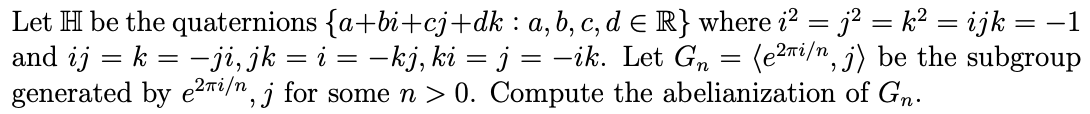Upper Level MathOpen questionpancho ono2022-10-25

##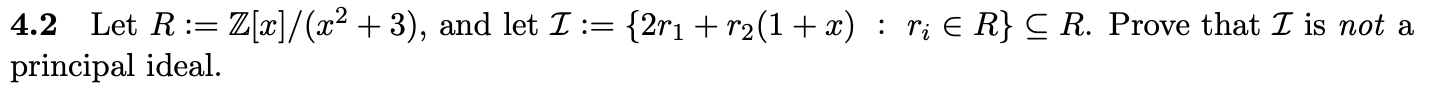Upper Level MathOpen questionAnyangwa Emmanuel Atekwana2022-10-16

## Write an equivalent first-order differential equation and initial condition for yy=−1+∫x1(t−y(t))dt

Upper Level MathOpen questionLindy Mandawe2022-10-15

## Is {8} ∈ {{8}, {8}}?

Upper Level MathOpen questionJUNSANG YOON2022-10-07

##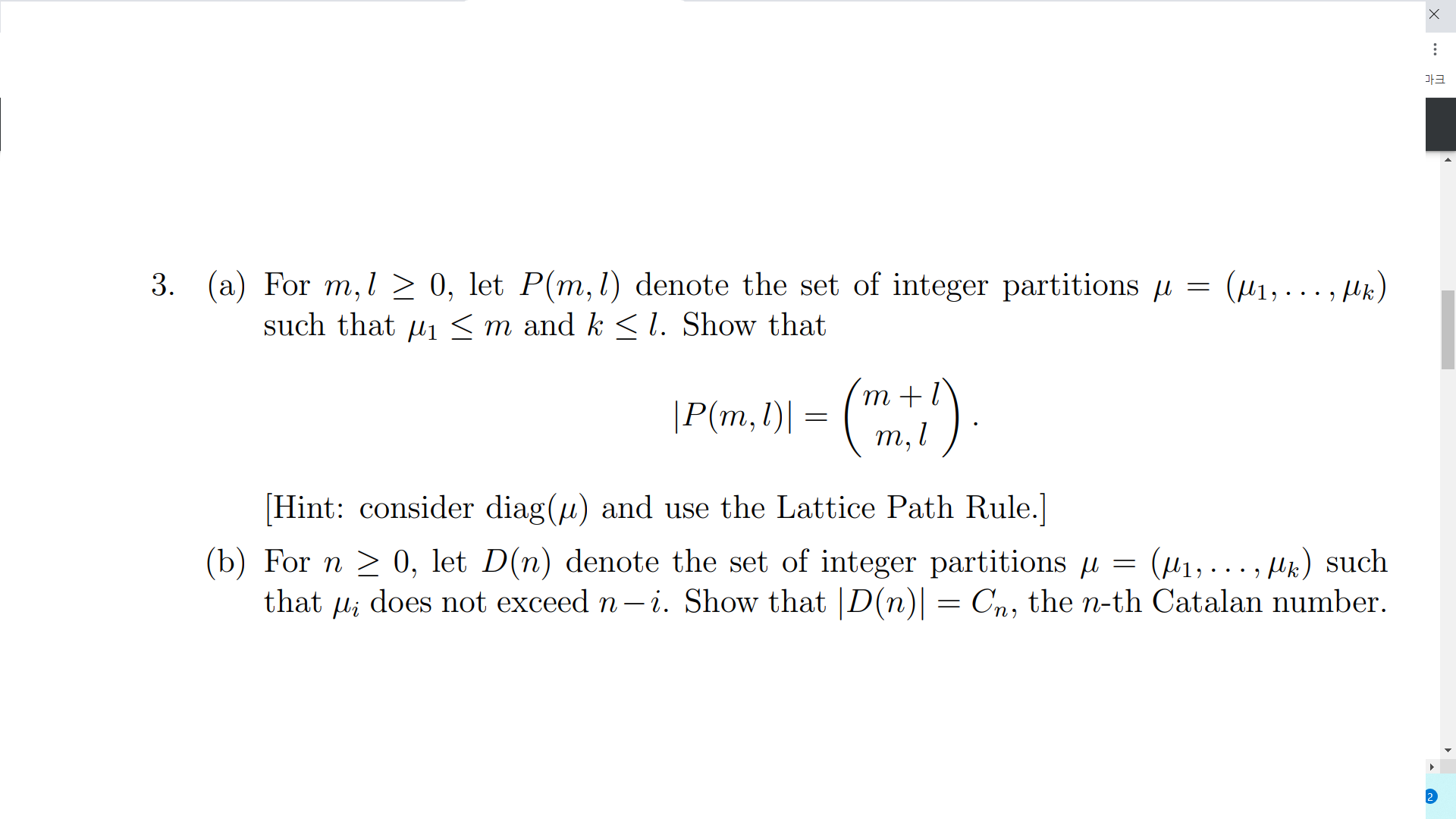Upper Level MathOpen questionAnokuhle Soganga2022-10-01

## $f\left(t\right)=t{e}^{-t}\mathrm{cos}h4t$

Upper Level MathOpen questionElizabet Rodriguez2022-09-28

## a. Write the formula for the volume V of a prism where B is the area of the base and h$h$ is the height.    V= b. Solve the formula for B$B$.    B= c. Use the new formula to find the area of the base of the prism.The area of the base of the prism is  square inches.

Upper Level MathOpen questionJolina Manos2022-09-24

## How many elements are in the set {3, 3, 3, 3}

Upper Level MathOpen questionorrocksydnie33 2022-09-22

## find the 52nd term of the arithmetic sequence -8,-12,-16

Upper Level MathOpen question2022-09-15

## 3y/4 - 4 = y/2 + 2

Upper Level MathOpen questionrennieb2018 2022-09-11

## what is the difference between a System of equations and an Equation?

In the majority of cases, students dealing with upper-level Math questions will have to solve not only numerical problems or equations but also verbal tasks. The answers that are provided below free of charge will help you to find solutions to your questions that are dealing with more advanced Maths problems. Remember that one must look into analysis and evaluation of original instructions, which is why upper-level Maths requires more thinking as you study questions and see how the answers have originated. The trick is to see similarities in solutions that are being provided as it always helps.